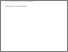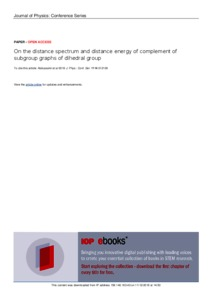# On the distance spectrum and distance energy of complement of subgroup graphs of dihedral group

Abdussakir, Abdussakir and Susanti, Elly and Turmudi, Turmudi and Jauhari, Mohammad Nafie and Ulya, Nanda M. (2018) On the distance spectrum and distance energy of complement of subgroup graphs of dihedral group. Journal of Physics: Conference Series, 1114 (1). pp. 1-6. ISSN 1742-6588Preview
Text (full text)
3841.pdf - Published Version

Full text available at: http://iopscience.iop.org/article/10.1088/1742-659...

## Abstract

Let G is a connected simple graph and V(G) = {v1, v2, ..., vp} is vertex set of G. The distance matrix of G is a matrix D(G) = [d ij ] of order p where [d ij ] = d(v i , v j ) is distance between v i and v j in G. The set of all eigenvalues of matrix D(G) together with their corresponding multiplicities is named the distance spectrum of G and denoted by spec D (G). The distance energy of G is ${E}_{D}(G)={\sum }_{i=1}^{p}|{\lambda }_{i}|$, where λi are eigenvalues of D(G). In the recent paper, the distance spectrum and distance energy of complement of subgroup graphs of dihedral group are determined.

Item Type: Journal Article 01 MATHEMATICAL SCIENCES > 0101 Pure Mathematics > 010104 Combinatorics and Discrete Mathematics (excl. Physical Combinatorics)01 MATHEMATICAL SCIENCES > 0101 Pure Mathematics > 010105 Group Theory and Generalisations Faculty of Mathematics and Sciences > Department of Mathematics Abdussakir Abdussakir 13 Dec 2018 09:35View Item